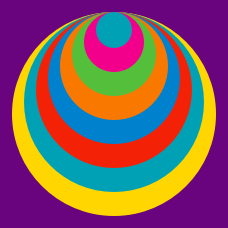Geometry

# Composite Figures - Solving with Algebra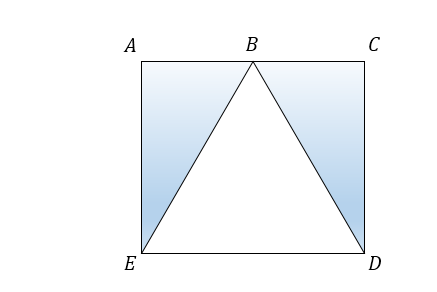If $ACDE$ is a rectangle and $B$ is a point on side $AC$ such that $\triangle BED$ is an equilateral triangle with side length $36,$ what is the area of the shaded region in the above diagram?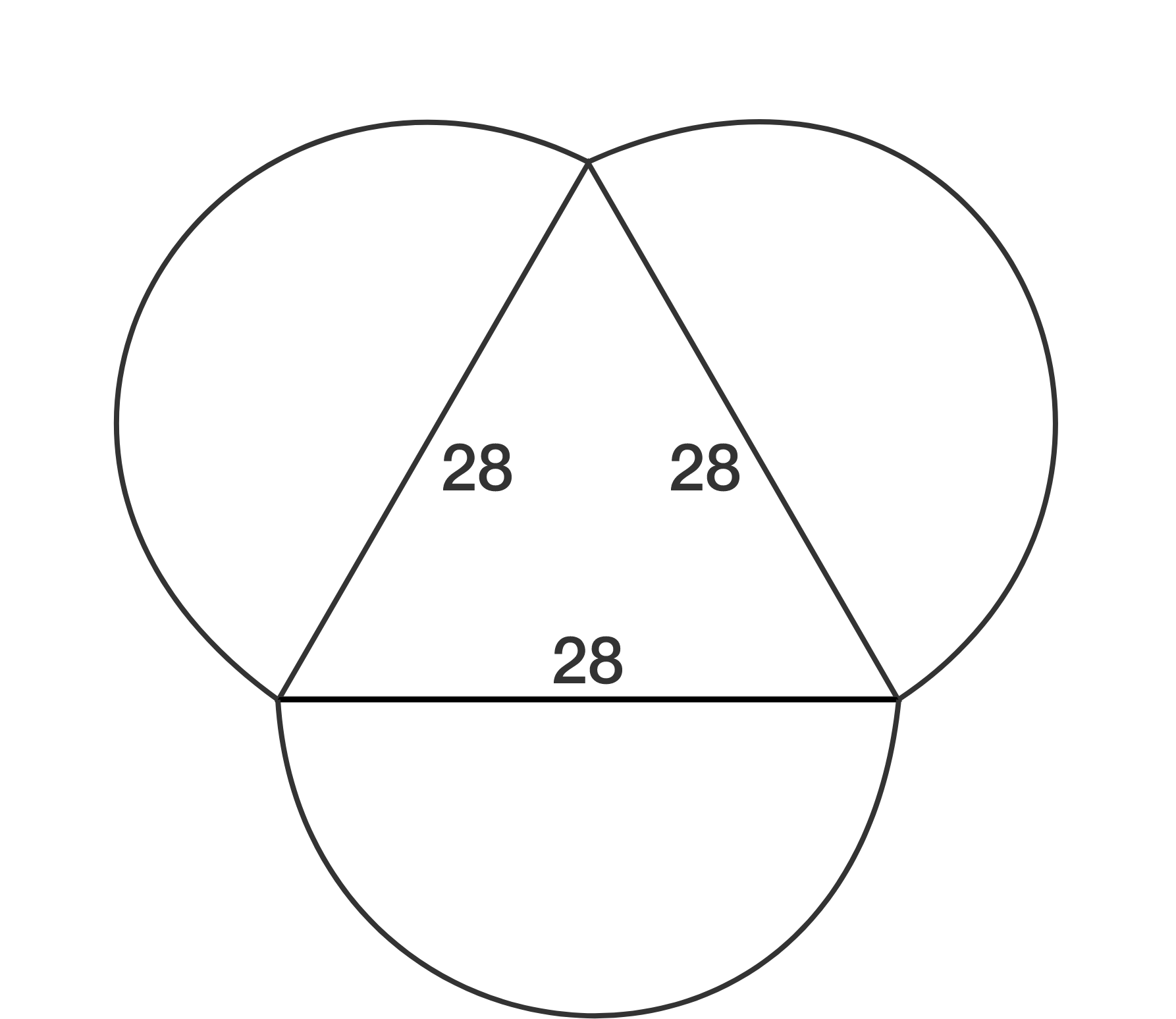On each of the edges of an equilateral triangle with side length 28, we construct a semicircle with diameter 28 that extends outwards. The area of the enclosed figure has the form $a \sqrt{b} + c \pi$, where $a, b,$ and $c$ are integers and $b$ is not divisible by the square of a prime. What is the value of $a+b+c$?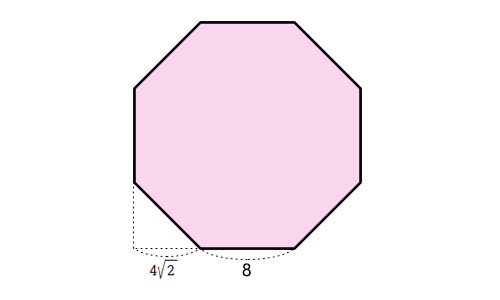If the side length of a regular octagon is $8$, what is the area of the octagon?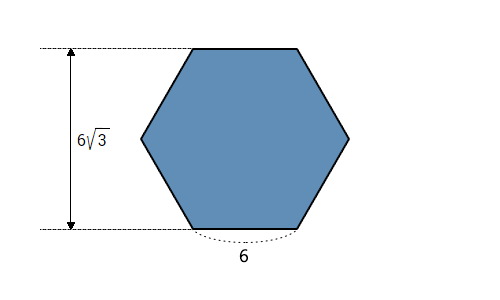If the side length of a regular hexagon is $6$, what is the area of the hexagon?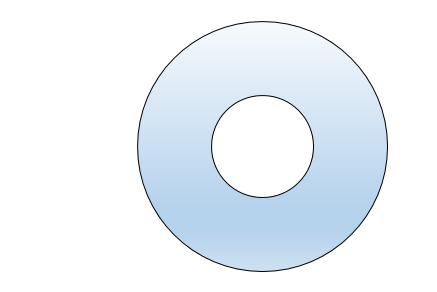What is the area of an annulus with inner radius $3$ and outer radius $8?$

×